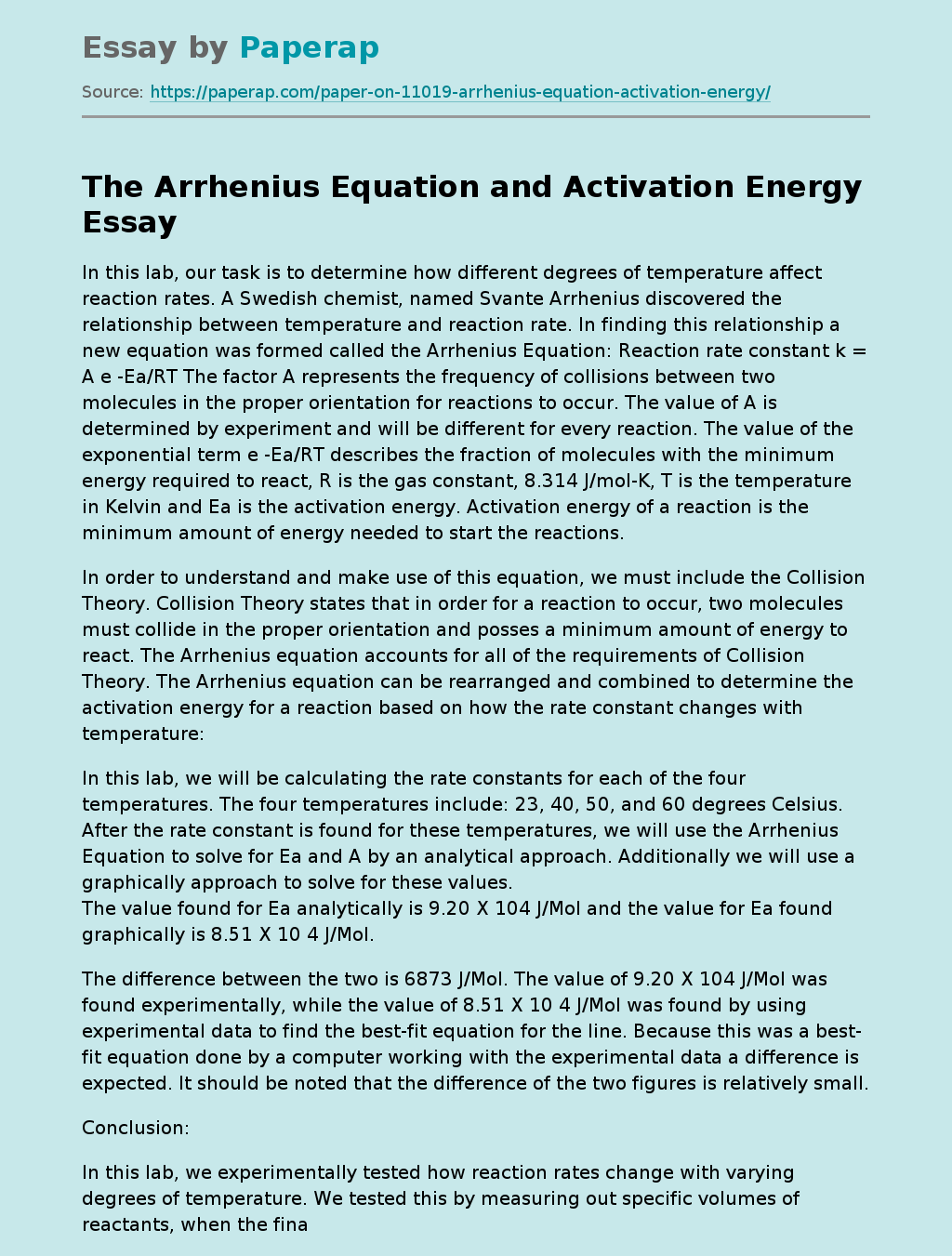# The Arrhenius Equation and Activation Energy

In this lab, our task is to determine how different degrees of temperature affect reaction rates. A Swedish chemist, named Svante Arrhenius discovered the relationship between temperature and reaction rate. In finding this relationship a new equation was formed called the Arrhenius Equation: Reaction rate constant k = A e -Ea/RT The factor A represents the frequency of collisions between two molecules in the proper orientation for reactions to occur. The value of A is determined by experiment and will be different for every reaction.

The value of the exponential term e -Ea/RT describes the fraction of molecules with the minimum energy required to react, R is the gas constant, 8.314 J/mol-K, T is the temperature in Kelvin and Ea is the activation energy. Activation energy of a reaction is the minimum amount of energy needed to start the reactions.

In order to understand and make use of this equation, we must include the Collision Theory. Collision Theory states that in order for a reaction to occur, two molecules must collide in the proper orientation and posses a minimum amount of energy to react.

The Arrhenius equation accounts for all of the requirements of Collision Theory. The Arrhenius equation can be rearranged and combined to determine the activation energy for a reaction based on how the rate constant changes with temperature:

In this lab, we will be calculating the rate constants for each of the four temperatures. The four temperatures include: 23, 40, 50, and 60 degrees Celsius. After the rate constant is found for these temperatures, we will use the Arrhenius Equation to solve for Ea and A by an analytical approach.

Get quality help nowKarrieWritesVerified

Proficient in: Change5 (339)

“ KarrieWrites did such a phenomenal job on this assignment! He completed it prior to its deadline and was thorough and informative. ”+84 relevant experts are online

Additionally we will use a graphically approach to solve for these values. The value found for Ea analytically is 9.20 X 104 J/Mol and the value for Ea found graphically is 8.51 X 10 4 J/Mol.

The difference between the two is 6873 J/Mol. The value of 9.20 X 104 J/Mol was found experimentally, while the value of 8.51 X 10 4 J/Mol was found by using experimental data to find the best-fit equation for the line. Because this was a best-fit equation done by a computer working with the experimental data a difference is expected. It should be noted that the difference of the two figures is relatively small. In this lab, we experimentally tested how reaction rates change with varying degrees of temperature. We tested this by measuring out specific volumes of reactants, when the final reactant was placed in the flask we started the stopwatch.

We were able to time the reaction visually by the change in color, once the reactant color turned clear the reaction was over and the stopwatch was stopped. We did this four times with the variable being the temperature of the water baths that the flasks were in. The first run was done with no water bath, thus at room temperature of 23ï¿½C and the other three runs at 40, 50 and 60ï¿½ C. When we solved for Ea1 the value was 8.94 X102 KJ/Mol this represents the two lowest temperature and the value of Ea2 was 9.45 X 101 KJ/Mol for the two highest temperatures.

Our data supports the idea that as temperature increases the rate increased, this was the true for all four runs. As with any experiment there is always a margin of error, in this case we were not working in a closed environment, and the fact that we took the flask out of the water baths before reading the final temperature occur twice which could possible provide an error. Prior to doing this lab, I have been told as well as have read that it is a clear understanding in the scientific world that a change of 10ï¿½C will double the rate of a reaction. We tested this in this lab, and once again this understanding has been substantiated. This experiment emphasized that the Arrhenius equation is a proving powerful tool for predicting reaction rates over a wide range of temperatures. After a final analysis of our data, I feel confident that our experiment was a success. My understanding of this concept is more firmly in place than prior to this experiment.

### References

1. Moore, John W., et al. The Chemical World Concepts and Applications. Orlando: Harcourt Brace & Company 1994.
2. Silberberg, Martin S., 2000. Chemistry: The Molecular Nature of Matter and Change. Third Edition. New York: McGraw-Hill Higher, 2000.